﻿ Mathematical Symbols And Problem Solving Stock Photo - Image of idea, creative: 124056176

# Mathematical symbols and problem solving.

## Math course book and math symbols on it. problem solution concept

More similar stock images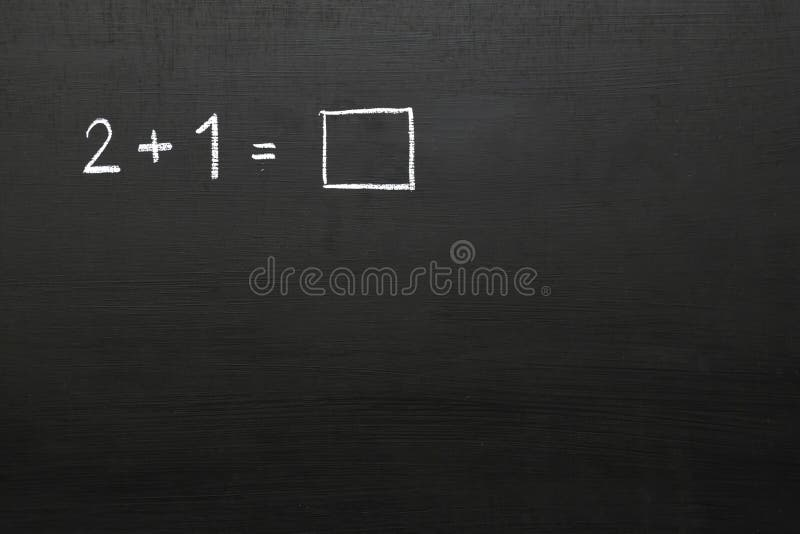Blackboard with Math problem. Numbers and mathematical symbols u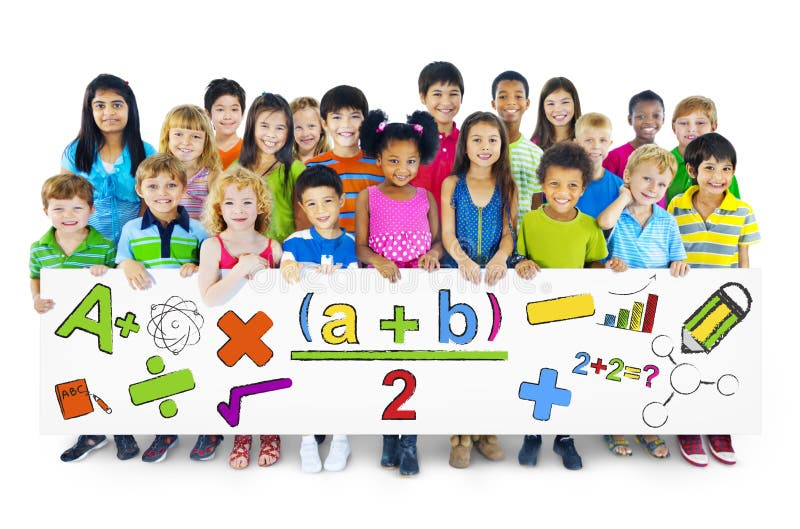Diverse Cheerful Children Holding Mathematical Symbols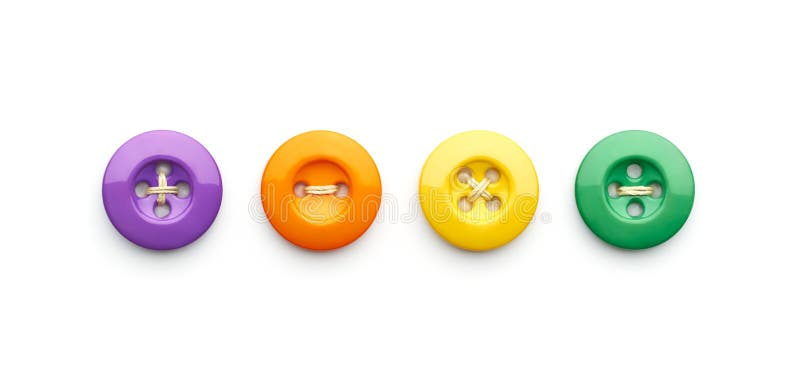Mathematical Symbols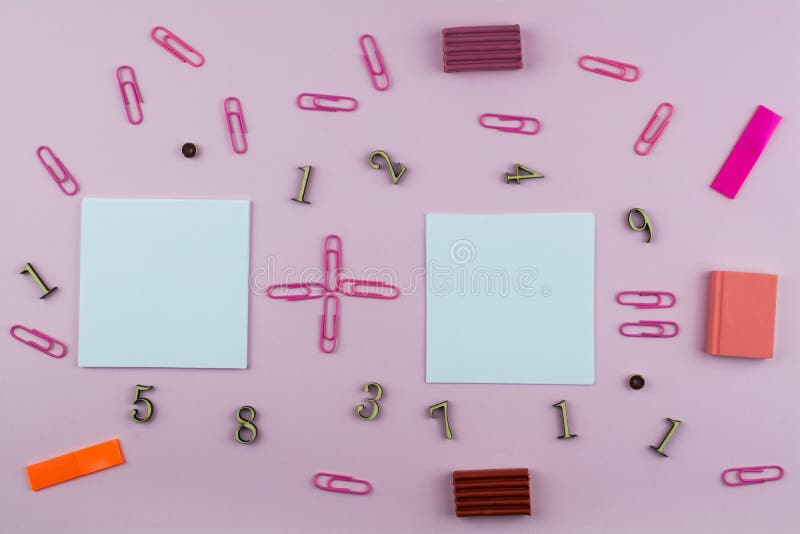Stationery for the school of pink and red color, members and mathematical symbols on a pink background. Space for text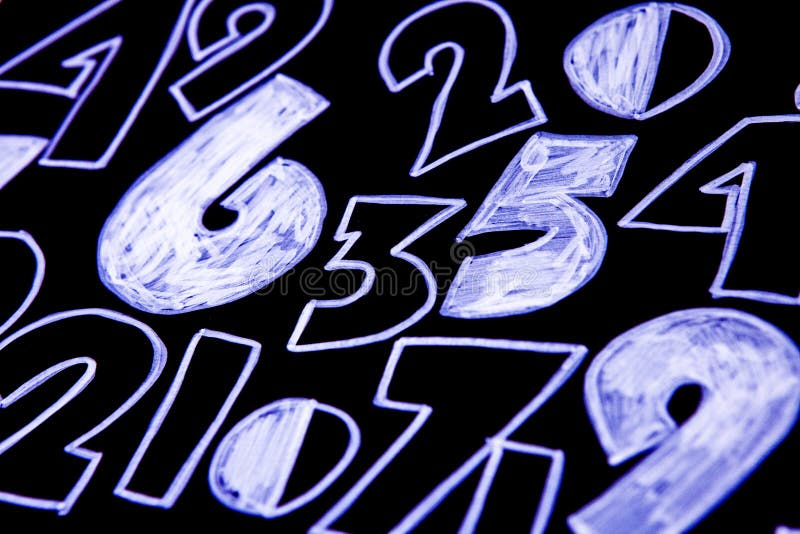Background of numbers. from zero to nine. Numbers texture. Currency symbols. Numerology. Mathematical equations and formulas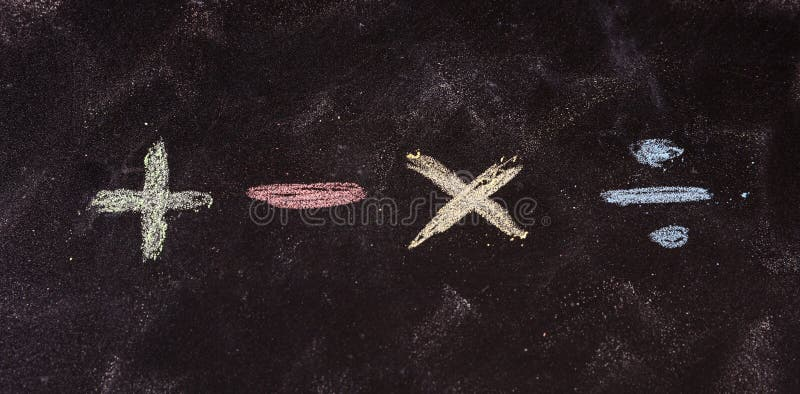Mathematical symbols written with colorful chalks, , on blackboard background.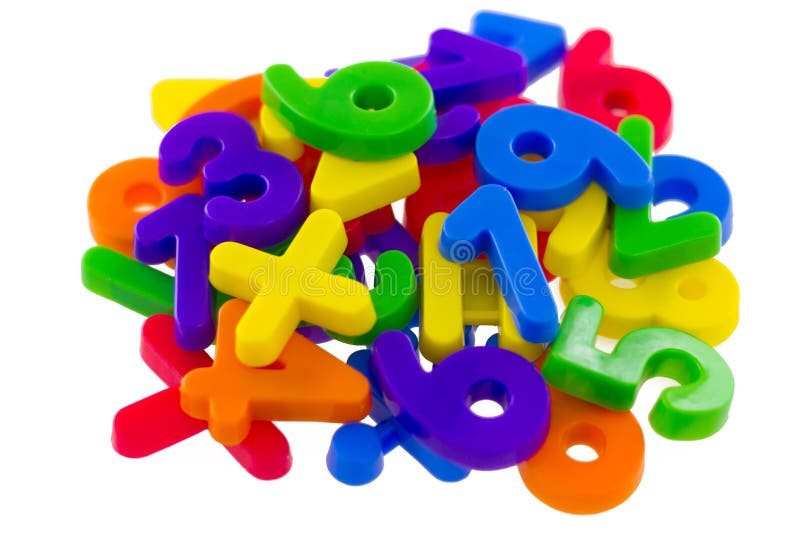Assorted Numbers and Mathematical Symbols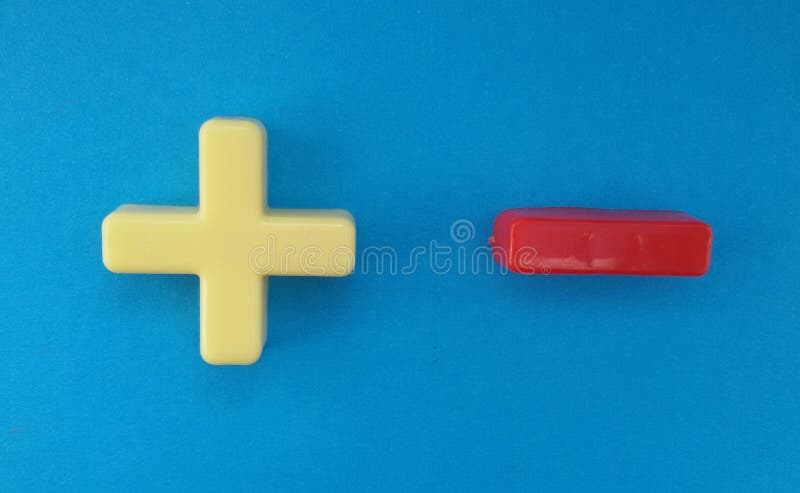Plus and minus signs over blue. Mathematical signs.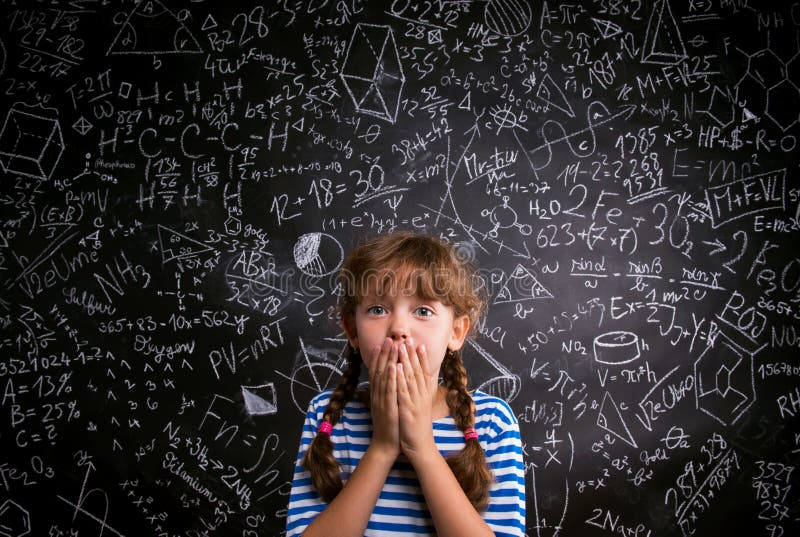Surprised girl, hands on mouth, blackboard with mathematical symbols
More stock photos from Osonmez2's portfolio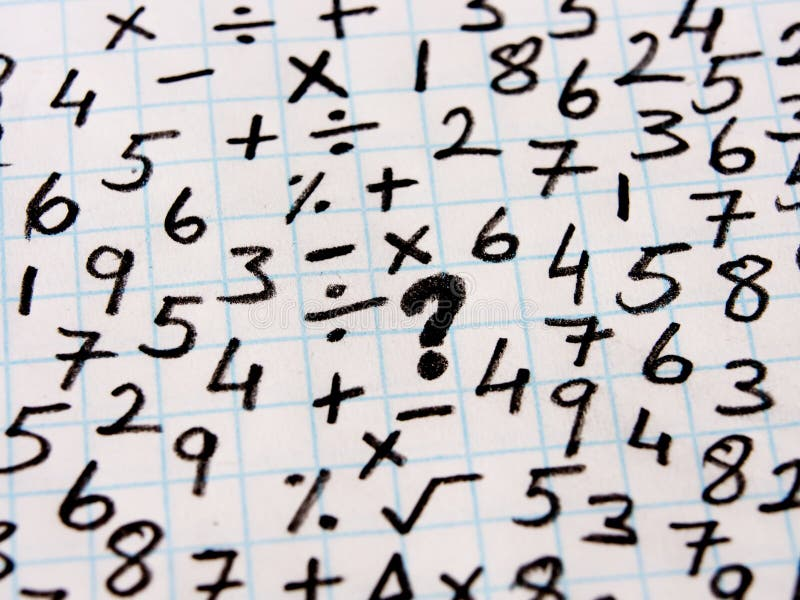Mathematical symbols and problem solving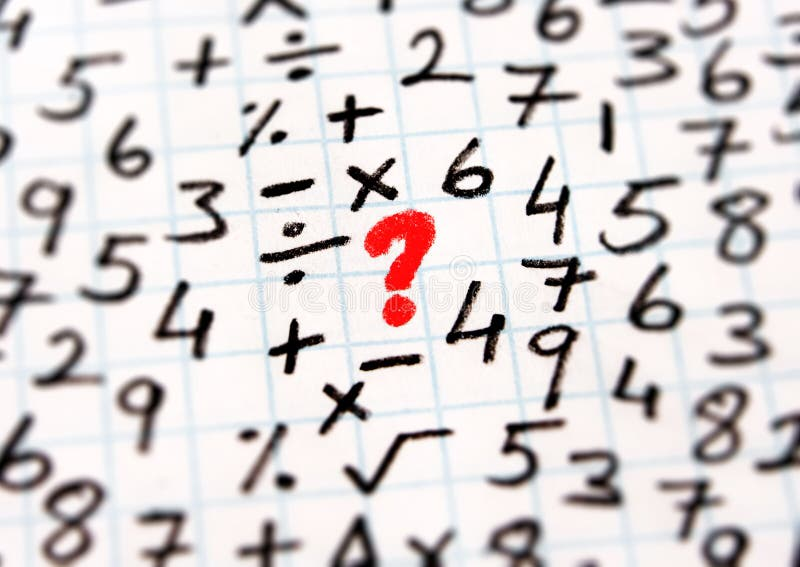Mathematical symbols and problem solving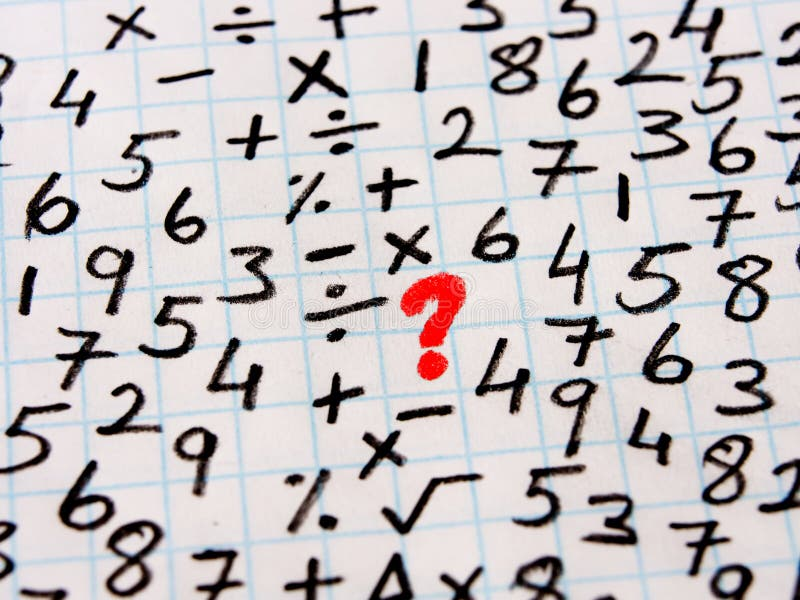Mathematical symbols and problem solving
Related categories